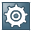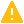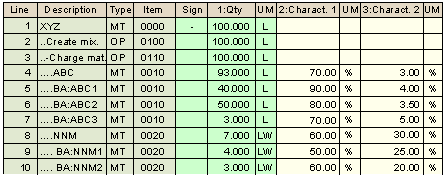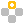Material Quantity Calculation With Batch DataUse

If you use materials that are subject to batch management, you may need to take quantities and properties of specific batches into account when your perform material quantity calculations in the process order. Depending on the active ingredient concentration of a component batch, you may need, for example, different quantities of another component, that is used as a filling material.

Since no batches are assigned in the master recipe, you must use the values planned in the material item when you perform calculations there. In the process order, however, you must perform the same calculation again after you have carried out batch determination due to the values of one, or in case of a batch split, several batches selected.

For this reason, SAP provides the functions described below to enable you to use the data of a material item in the master recipe and the data of subordinate batches after the batch determination in the process order. These functions can be used in addition to the functions described in Basic Functions of Material Quantity Calculation.Material quantity calculation is not automatically performed after batch determination in the order.

Integration

• If you use batch data to calculate component quantities, note the following when you carry out batch determination in the process order:
• You must first carry out batch determination for the components to whose data you refer in the formulas for other components.
• Material quantity calculation is not automatically performed after batch determination. If required, you must start the calculation manually to update the data of dependent components.
• If you also want to use active ingredient management of the Batch Management component, you can perform material quantity calculation either for the physical quantity or for the active ingredient proportion of a component.

If you use active ingredient proportions, you can use exact active ingredient quantities to plan the quantity structure of your orders.

LO - Batch Management

Prerequisites

To be able to use material quantity calculation in a master recipe, you must have defined a production version for the master recipe.

To transfer the data from material quantity calculation from a master recipe to the process order, you must create the process order with the corresponding production version.

For you to be able to take into account batch properties such as the active ingredient potency in material quantity calculation, the following requirements must be met:

• It must be possible to enter the property as a numeric value.
• You must classify the material master record, the BOM item or the material item of the process order as well as the batch with a class of class type Batch.
• You must assign the batch property as a characteristic to this class and assign a value to it in the batch as well as in the material master record, the BOM item, or the material list of the process order.

Material quantity calculation uses the following values:

• In the master recipe, the value from the BOM or, if this value is missing, the value from the material master record
• In the process order, the value from the material list of the order

If multiple values or value ranges have been defined, the system uses the lowest value.In the material master record, you can also enter a planned value for the batch characteristic for active ingredient management (Additional data, Proportion/prod.unit tab page). To avoid inconsistencies between active ingredient management and material quantity calculation, we recommend using the lowest characteristic value from material classification.

Features

The following sections provide an overview of the functions of material quantity calculation that take the batch data of a material component into account. In the expressions used:

• <Material item> stands for the line number of the material item
• <Characteristic column> stands for the column number of the batch characteristic

The examples refer to the following data:Determining the Number of Batches in a Material Item

Use the following function:

CNUM(<material item>)

The function determines the number of batches that belong to a material item. As long as no batch has been assigned to the material item, the function has value 1.CNUM(004) = 3

Adding up Batch Quantities or Characteristic Values

This function adds up the batch quantities or the values in the characteristic column you specified for all batches of a material item. As long as no batches have been assigned to the material item, the function returns the quantity of the material item or the characteristic value of the material item.

Use the following function:

• To add up batch quantities, use:

CSUM(<material item>)CSUM(004) = 40 + 50 + 3 = 93

• To add up characteristic values, use:

CSUM(<material item>;<characteristic column>)CSUM(004;002) = 90 + 80 + 70 = 240

Calculating the Arithmetic Mean of Batch Quantities or Characteristic Values

This function calculates the arithmetic mean of the batch quantities or the characteristic column you specified from all batches of a material item. As long as no batches have been assigned to the material item, the function returns the quantity of the material item or the corresponding characteristic value of the material item.

Use the following function:

• To calculate the arithmetic mean of the batch quantities, use:

CAMV(<material item>)CAMV(004) = (40 + 50 + 3) / 3 = 31

• To calculate the arithmetic mean of the characteristic value, use:

CAMV(<material item>;<characteristic column>)CAMV(004;002) = (90 + 80 + 70) / 3 = 80

Adding up Weighted Batch Quantities or Characteristic Values: Product Sum

For each batch of a material item, this function multiplies the batch quantity by the value of the characteristic column you specified or the values of both characteristic columns specified. It then adds up the results. The system interprets the characteristic value that you use to weight the first value as a percentage if the description of its unit contains a percent sign (%).

As long as no batches have been assigned to the material item, the function calculates the product of the quantity and characteristic value of the material item or the product of the two characteristic values of the material item.

You may need this function, for example, to calculate the total of the active ingredient quantities from physical batch quantities based on the active ingredient concentration. Use the following function:

• To calculate the product sum of batch quantities, use:

CPSM(<material item>;<characteristic column>)CPSM(004;002) = (40 * 0.9) + (50 * 0.8) + (3 * 0.7) = 78.1

• To calculate the product sum of characteristic values, use:

CPSM(<material item>;<characteristic column 1>;<characteristic column 2>)CPSM(004;002;003) = (90 * 0.04) + (80 * 0.035) + (70 * 0.05) = 9.9

Adding up Weighted Batch Quantities or Characteristic Values: Quotient Sum

For each batch of a material item, this function divides the batch quantities by the value of the characteristic column you specified or the value of the first characteristic column by that of the second column. It then adds up the results. The system interprets the characteristic value that you use to weight the first value as a percentage if the description of its unit contains a percent sign (%).

As long as no batches have been assigned to the material item, the function divides the quantity of the material item by the corresponding characteristic value or the first characteristic value of the material item by that of the second value.

You may need this function, for example, to calculate the total of the physical batch quantities from the active ingredient quantities of batches based on the active ingredient concentration. Use the following function:

• To calculate the quotient sum of batch quantities, use:

CQSM(<material item>;<characteristic column>)CQSM(008;002) = (4 / 0.5) + (3 / 0.6) = 13

• To calculate the quotient sum of characteristic values, use:

CQSM(<material item>;<characteristic column 1>;<characteristic column 2>)CQSM(008;002;003) = (50 / 0.25) + (60 / 0.2) = 500

Examples